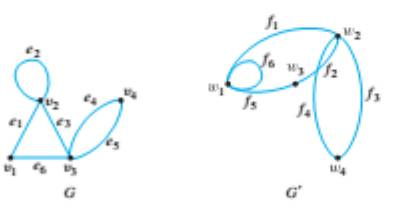Chapter 10.3, Problem 3ES### Discrete Mathematics With Applicat...

5th Edition
EPP + 1 other
ISBN: 9781337694193

#### Solutions

Chapter
Section### Discrete Mathematics With Applicat...

5th Edition
EPP + 1 other
ISBN: 9781337694193
Textbook Problem
4 views

# For each pair of graphs G and G’ in 1-5, determine whether G and G’ are isomorphic. If they are, give functions g: V(G) → V(G’) and h:E(G) → E(G’) that define the isomorphism. If they are not, give an invariant for graph isomorphism that they do not share.To determine

Whether G and G are isomorphic. If they are, give functions g:V(G)V(G) and h:E(G)E(G) that define the isomorphism. If they are not, give an invariant for graph isomorphism that they do not share.

Explanation

Given information:

Calculation:

Two graphs are G and G’ (with vertices V ( G ) and V ( G’ ) respectively and edges E ( G ) and E ( G’ ) respectively) are isomorphic if there exists one-to-one correspondence

g:V(G)V(G) and h:E(G)E(G) such that v is an endpoint of eg(v) is an endpoint of h(e).

V(G) are all vertices of the graph GV(G)are all vertices of the graph GE(G) are all edges of the graph GE(G) are all edges of the graph G

V(G)={v1,v2,v3,v4}V(G')={w1,

### Still sussing out bartleby?

Check out a sample textbook solution.

See a sample solution

#### The Solution to Your Study Problems

Bartleby provides explanations to thousands of textbook problems written by our experts, many with advanced degrees!

Get Started